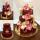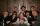# Stamps 2

Dennis spent 34.15 on stamps. The number of .56 is 10 less than four times of stamps bought for .41. How many of each stamp did he buy?

Result

a =  50
b =  15

#### Solution:

0.56a + 0.41b = 34.15
a = 4b-10

0.56a+0.41b = 34.15
a-4b = -10

a = 50
b = 15

Calculated by our linear equations calculator.

Leave us a comment of example and its solution (i.e. if it is still somewhat unclear...):

Showing 0 comments:Be the first to comment!#### To solve this example are needed these knowledge from mathematics:

Do you have a system of equations and looking for calculator system of linear equations?

## Next similar examples:

1. Two numbersWe have two numbers. Their sum is 140. One-fifth of the first number is equal to half the second number. Determine those unknown numbers.
2. TheatroTheatrical performance was attended by 480 spectators. Women were in the audience 40 more than men and children 60 less than half of adult spectators. How many men, women and children attended a theater performance?
3. EurosPeter, Jane and Thomas have together € 550. Tomas has 20 euros more than Jane, Peter € 150 less than Thomas. Determine how much has each of them.
4. DollarsMama split 760 dollars Jane, Dane and Eva as follows: Jane got three times more than Dane and Dane and got 40 more than Eva. How much does get each of them?
5. Money duoJulius and Mark have together 45 euros. Mark has 50% more money than Julius. Determine the amount of money that have Mark and Julius.
6. Three brothersThe three brothers have a total of 42 years. Jan is five years younger than Peter and Peter is 2 years younger than Michael. How many years has each of them?
7. MushroomsEva and Jane collected 114 mushrooms together. Eve found twice as much as Jane. How many mushrooms found each of them?
8. TreesAlong the road were planted 250 trees of two types. Cherry for 60 CZK apiece and apple 50 CZK apiece. The entire plantation cost 12,800 CZK. How many was cherries and apples?
9. Tour35 people went on a tour and paid 8530, -. Employees pay 165, and family members 310. How many employees and how many family members went to the tour?
10. Linear systemSolve this linear system (two linear equations with two unknowns): x+y =36 19x+22y=720
11. Equations - simpleSolve system of linear equations: x-2y=6 3x+2y=4
12. Hotel roomsIn the 45 rooms, there were 169 guests, some rooms were three-bedrooms and some five-bedrooms. How many rooms were?
13. EquationsSolve following system of equations: 6(x+7)+4(y-5)=12 2(x+y)-3(-2x+4y)=-44
14. Sweets3 chocolate and 7 cakes cost 85, - CZK. 2 chocolates and 6 cakes cost 86, - CZK. How much is 5 chocolates and 9 cakes? I wonder how to get the result, but only by logic without the use of a system of equations
15. BanknotesEva deposit 7800 USD in 50 banknotes in the bank. They had value 100 USD and 200 USD. How many were they?
16. SistersSisters Janka and Danka are saved together 220 CZK. On a trip to Janka wants to take a fifth of her savings and Danka quarter. Then they will have 50 CZK on the trip. How much money saved Janka and Danka?
17. 12 apples12 apples and 2 loaves of bread cost 5.76 and 6 apples and 3 loaves of bread cost 7.68. How much is a loaf of bread?